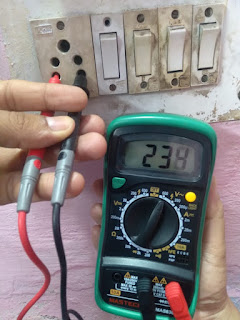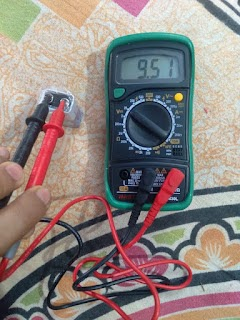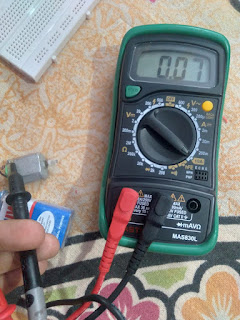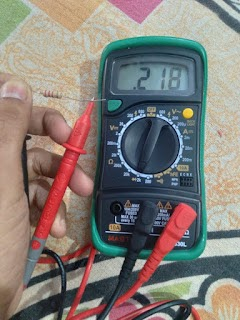Multimeter is an electronic measuring instrument by which we can measure resistance,  AC Voltage, DC voltage and current. Beside this we can also test elements like Capacitor, diode, transistor  and continuity of a circuit. Companies of multimeter for home use and learning are mastech, fluke, rishabh etc. These multimeter are available from 800 to 10000 rupees depending upon range of multimeter.

#### How to use multimeter

Multimeter has three terminals voltage/resistance, current and common. There are two probes with multimeter i.e. one red and other black. Insert black probe in common terminal and red probe in Voltage terminal to check the voltage or resistance. To check current, connect red probe in current terminal. While testing any physical quantity ensure you have selected corresponding range of that physical quantity. For example, if you are measuring 230 V AC voltage put selector rotation knob to voltage range more than 230 V AC range for proper and accurate reading and also for safe working of multimeter otherwise multimeter can damage soon. I am using a Mastech company made multimeter to check physical quantities.

Lets start to check all physical quantities one by one :-

1. AC Voltage measurement :- I am measuring my room supply voltage so to check it. First connect black probe in common terminal and red probe in Voltage terminal as shown in Fig. 1. As we know supply for 1 kW meter is 230 V AC that is why I am putting selector rotating switch in 600 V range. You can see in image reading of multimeter voltage which is 234 V.Fig. 1. AC voltage measurement using multimeter.

2. DC Voltage measurement:- To check DC voltage of a 9V battery, I am using same connection but putting selector switch in 20 V DC range. Multimeter reading is 9.51 V as shown in Fig. 2.Fig. 2. DC voltage measurement using multimeter.

3. Current measurement:- To measure current, I am using a small DC motor. Connect multimeter similarly put selector knob in DC current range of 10A. Reading of current is 0.07 Ampere as shown in Fig. 3.Fig. 3. DC current measurement using multimeter.

4. Resistance Measurement:- Connect red probe in resistance terminal and black probe in common. Select resistance range according to resistance value as I am measuring 220 ohm resistance so I am selecting 2k ohm range. Multimeter reading is .218 K ohm or 218 E / Ohm as shown in Fig. 4.Fig. 4. Resistance measurement using multimeter.

Author: Amarjeet Singh Jamwal authored articles on Basic Electronics EngineeringElectric Traction, and Electronics Practical for INFO4EEE Website.